﻿ The Linearized Fourier Thermal Model Applied to Au Nanoparticles 1D and 2D Lattices under Intense Nanoseconds Laser Irradiation Pulses

# The Linearized Fourier Thermal Model Applied to Au Nanoparticles 1D and 2D Lattices under Intense Nanoseconds Laser Irradiation Pulses

Mihai Oane*, Ion N Mihăilescu and Bogdan Sava
National Institute for Laser, Plasma and Radiation Physics, Str. Atomiştilor 409, Măgurele, 077125, Romania
*Corresponding Author: Mihai Oane, National Institute for Laser, Plasma and Radiation Physics, Str. Atomiştilor 409, Măgurele, 077125, Romania, Tel: +40 0771-408-318, Email: [email protected]

Received Date: Feb 12, 2019 / Accepted Date: Feb 22, 2019 / Published Date: Feb 28, 2019

### Abstract

Very recently, new exact analytical solutions of the Fourier heat equation have been proposed by Zhukovsky. Since the Zhukovsky solutions are very powerful we applied the Zhukovsky formalism to a specific experimental situation, i.e. to a one dimensional (1D) lattice composed of Au nanoparticles of radius 20 nm in water media, under 20 ns laser pulse irradiation. In addition, we calculated the thermal field in the 2D spatial dimensions case for a single Au nanoparticle in water irradiated under the same conditions but with a different fluence. These results exemplify how the new Zhukovsky formalism contributes to the real physical view of such laser thermalized processes. This new theoretical approach could be easily extended to laser processing in general, and laser additive manufacturing in special.

Keywords: Au nanoparticles in water; Fourier-Zhukovsky thermal model

#### Introduction

In the present paper we study some problems related to heat transport using the Fourier heat differential equations , which describe a wide range of physical processes. A parallelism between Zhukovsky solutions [2-4] and the integral transform technique (ITT) [5-7] is suggested. The thermal fields of nanoparticles under laser irradiation deduced using the ITT [5-7] was studied in reference .

#### The Mathematical Formalism

In reference  the following heat equation is considered: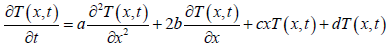(1)

Here T is the temperature, x is the spatial coordinate, t is the time parameter and a, b, c and d are real constants unit less. First we set: b=c=0, a=d=1 and obtain the simplified form of the heat equation: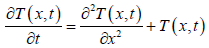(2)

The ITT boundary condition T(x,0) corresponds to the heat source term in the Fourier-Zhukovsky model i.e.:

T(x,0)=δ (x) (3)

After some algebraic manipulations, we obtain the following solution for eqn. (2) with the boundary condition (3):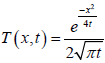(4)

Considering the case of the thermal field produced by laser irradiation of a nanoparticle-cluster , we have the following source term of a given nanoparticle (p) in a particles linear 1D lattice the following expression: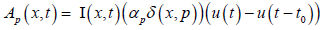(5)

where I (x,t) is the incident laser intensity, u is the step function, αp is the optical absorption coefficient of the particle and t0 is the irradiation exposure time i.e., the laser pulse duration .

In the specific typical case of a constant spatio-temporal laser irradiation intensity I (x,t)=I0, the source term for a 1-D lattice of nanoparticles system is given by: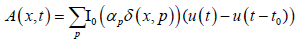(6)

where the sum is over all p nanoparticles of the 1-D lattice.

One may observe that the heat source terms from both formalisms (Zhukovsky and integral transform technique - ITT) are the same up to a given constant. As a consequence we can consider the source term of ITT as the Fourier-Zhukovsky thermal parameter h in eqn. (3).

#### Simulations for the 1D Model

In the present chapter we deal with simulations regarding the thermal model applied to a 1D lattice of nanoparticles under a laser flash of irradiation using the Fourier-Zhukovsky thermal model. From the above discussion it is clear that the boundary condition (3) represents “the heat source term” from the standard heat equation. In order to be able to plot the thermal fields, we use the following delta Dirac equation: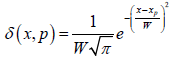(7)

Here W is a parameter which gives the strength of interaction (the interaction parameter being the absorption coefficient) and xp is the position of the particle p. Let us consider a nanoparticle under the ultra-short laser irradiation time: tpulse=20 ns and fluence of F=2J/cm2. The laser beam is incident perpendicularly onto the nanoparticles 1D lattice. First we observe the thermal field increment of a nanoparticle with radius of 20 nm at x1=0, as shown in Figure 1.

Figure 1: The thermal field given by Fourier-Zhukovsky model for a 20 ns pulse laser heating of the nanoparticle positioned at x1=0 with b=c=0, a=d=1.

In Figure 2 we show a nanoparticle thermal field at a different position: xp=50 nm. In Figure 3 we simulate the thermal distribution for the whole 1-D lattice, formed from three nanoparticles at the coordinates xp=-50 nm, xp=0 nm and xp=50 nm.

Figure 2: The thermal field given by Fourier-Zhukovsky model for 20 ns duration of laser pulsed heating of a nanoparticle positioned at xp=50 nm with b=c=0, a=d=1.

Figure 3: The thermal field given by the Fourier-Zhukovsky model for a pulsed laser heating of a 1-D lattice of nanoparticles, positioned at xp=0, xp=50 nm and xp=-50 nm with b=c=0, a=d=1)

If we analyze Figures 1-3 we observe that for a given particle p of radius 20 nm, the thermal field is maximum at the centered position xp and decreases along the radius direction. One can notice that as the distance between xp and x increases the temperature decreases. It is important to notice also that at the boundary between the Au nanoparticle and the water media the temperature is different from zero. In conclusion the nanoparticle transfers a part of the heat to the water ; which for a single particle case goes to zero at about 120 nm distance from the center of the nanoparticle. If we analyze Figure 3, we can observe that the temperature increases drastically along the 1D lattice for the case of three Au nanoparticles from which we conclude that we have a superposition of the thermal fields from the individual particles. We may also observe from Figure 3 that is possible, to have an increase of the temperature which is higher than the Au melting point.

For the case d # 1(b=c=0, a=1) we have the following solution for the eqn. (1):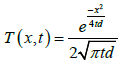(8)

For instance, we consider the results obtained in Figures 4-6 for: d=5 (b=c=0, a=1), and plot also the thermal field for the nanoparticles positioned at xp=0, and xp=50 nm. In Figure 6 we plot the overall result which is the superposition of the thermal distributions for 3 particles located at xp=0, xp=50 nm and xp=-50 nm. We used a fluence of F=0.2 J/cm2 for the Figures 4-6. We observe a continuous decrease of the temperatures and an almost zero value where the thermal fields interfere.

Figure 4: The thermal field given by Fourier-Zhukovsky model for a laser pulsed heating during 20 ns of a nanoparticle positioned at xp=0 with d=5.

Figure 5: The thermal field resulting from the Fourier-Zhukovsky model for a laser pulsed heating of 20 ns, of a nanoparticle positioned at xp=50 nm with d=5.

Figure 6: The thermal field given by Fourier-Zhukovsky model for a laser pulsed heating of 20 ns duration in a 1-D lattice of 3 nanoparticles, positioned at xp=0 nm, xp=50 nm, xp=-50 nm, with d=5.

The thermal field obtained by the Fourier-Zhukovsky model for a laser pulsed heating in the casen when there is a very high heat transfer coefficient at the same incident fluence is presented in Figure 7. A detailed discussion about the influence of the heat transfer coefficient is given in reference .

Figure 7: The thermal field given by Fourier-Zhukovsky model for a laser pulsed heating for the case of a material with very high heat transfer coefficient.

In Figure 8 we present the thermal field of the same irradiation conditions but for only a single Au nanoparticle, with radius of 10 nm. We push here the theory to its limits because 20 nm is usually regarded as the upper theoretical limit still usable with the Fourier equation .

Figure 8: The thermal field given by Fourier-Zhukovsky model for a laser pulse heating in the spatial limit of the Fourier equation applicability e.g.: 10 nm.

#### Simulations for 2D Particles Lattice Model

In the two dimensions model we have the following heat equation :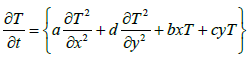(9)

If we set: T (x, y, 0)=δ (x, y); a=d ≠ 0 and b=c=0, we have the solution: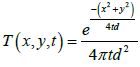(10)

The 2D case plot of the temperature distribution for a one nanoparticle under laser irradiation is given in Figure 9.

Figure 9: The simulated thermal field produced by a laser pulsed nanoparticle centered at (0, 0) using the “Fourier-Zhukovsky” model.

#### Discussions

We have plotted the thermal fields using the “Fourier-Zhukovsky” formalism for various values of the parameter “d” using eqn. (1). Analyzing Figures 1-6 we observed that the greater is d the lower is the temperature of the 1D lattice of nanoparticles. This observation leads us to the assumption that the term “d” is similar to the heat transfer coefficient H in the ITT-integral transform technique.

Our 1D and 2D results are in accordance with other thermal models, such as the example given in reference . Comparing to the theory described in reference [12-16], our model has the advantage that it can produce 3D graphs, showing the temperature as a function of both space and time. We are also dealing with short laser pulses of the order of ns magnitude. In other words our model is valid providing the absorption law is described correctly by a Dirac Delta function. We have thus realized two main achievements in the present paper: i) we have introduced a new thermal exact theoretical analytical model; namely: “Fourier-Zhukovsky” and ii) we arrived to the conclusion that in high vacuum conditions when we irradiate a 1D lattice of nanoparticles with ns intense laser beam pulses we observe the phenomena of the thermal field superposition from one nanoparticle onto the another. When we do not use high vacuum conditions the superposition leads to a drastic thermal field amplitude decrease.

#### Conclusions And Outlook

In the present paper we considered a parallelism between the very new formalism: “Fourier-Zhukovsky” for solving the heat equation and the “old” integral transform technique (ITT). We have applied the “Fourier-Zhukovsky”, to a 1-D lattice formed by three nanoparticles with a very high absorption coefficient. The key point of our comparison between the two models was given by the fact that the heat source term in the model “Fourier-Zhukovsky”, (given by the boundary condition T (x, 0)=δ (x) it is the same as the heat source term (A). In comparison with other models our proposed model has the advantage that it can resolve 3D thermal fields and does not require a too complicated formalism.

In conclusions we showed here that the “Fourier-Zhukovsky” is compatible with the integral transform technique (ITT) and it is indeed an excellent tool for calculating the thermal fields of individual nanoparticles arranged in a 2D model or 1D lattice interacting with ns-short laser pulses.

This approach can be useful for thermal fields simulations of carbon nanoparticles embedded in a vitreous matrix activated by laser pulses and electrical fields.

This new theoretical approach could be easily extended to laser processing in general, and laser additive manufacturing in special.

#### References

Citation: Oane M, Mihăilescu IN, Sava B (2019) The Linearized Fourier Thermal Model Applied to Au Nanoparticles 1D and 2D Lattices under Intense Nanoseconds Laser Irradiation Pulses. J Material Sci Eng 8: 513. DOI: 10.4172/2169-0022.1000513

Copyright: © 2019 Oane M, et al. This is an open-access article distributed under the terms of the Creative Commons Attribution License, which permits unrestricted use, distribution, and reproduction in any medium, provided the original author and source are credited.

Select your language of interest to view the total content in your interested language

##### Recommended Journals
Viewmore
• ###### 18th International Conference on Emerging Materials and Nanotechnology

February 26-27, 2020 London, UK

• ###### European Meeting on Materials Science and Nanotechnology

March 09-10, 2020 Rome, Italy

• ###### World Congress on Carbon and Advanced Energy Materials

March 16-17, 2020 Sydney, Australia

• ###### World Congress on Materials, Nanomaterials and Biobased Chemistry

April 20-21, 2020 London, UK

• ###### 36th World Congress on Materials Science and Nanotechnology

May 13-14, 2020 Tokyo, Japan

• ###### 20th International Conference on Materials Science and Engineering

May 25-26, 2020 Osaka, Japan

Viewmore
###### Article Usage
• Total views: 859
• [From(publication date): 0-0 - Dec 15, 2019]
• Breakdown by view type
• HTML page views: 805Can't read the image? click here to refresh